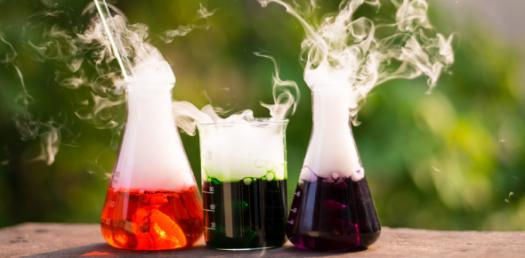# Chemistry Quiz: Can You Pass This Chemical Reaction Test?

Approved & Edited by ProProfs Editorial Team
At ProProfs Quizzes, our dedicated in-house team of experts takes pride in their work. With a sharp eye for detail, they meticulously review each quiz. This ensures that every quiz, taken by over 100 million users, meets our standards of accuracy, clarity, and engagement.
P
Community Contributor
Quizzes Created: 19 | Total Attempts: 59,849
Questions: 10 | Attempts: 3,410Settings.

• 1.

### A true solution is a homogeneous mixture of solute and solvent. Chalk powder in water is a heterogenous mixture. Is it a true solution?

• A.

No. it is not a true solution. It is called Suspension.

• B.

Yes. it is a true solution..

• C.

No. it is not a true solution. It is called Colloid.

• D.

No. it is a true solution.

A. No. it is not a true solution. It is called Suspension.
Explanation
The given explanation is correct. A true solution is a homogeneous mixture where the solute particles are evenly distributed throughout the solvent. In the case of chalk powder in water, the particles of chalk are not evenly distributed and can be seen as a separate phase, making it a heterogeneous mixture known as a suspension. Therefore, it is not a true solution.

Rate this question:

• 2.

### Solution that contains water as the solvent is called aqueous solution. If carbon disulphide is a solvent in a given solution, then the solution is called ______

• A.

Aqueous solution

• B.

Non aqueous solution

B. Non aqueous solution
Explanation
If carbon disulphide is the solvent in a given solution, then the solution is called a non-aqueous solution. Aqueous solutions are those that contain water as the solvent, while non-aqueous solutions are those that have solvents other than water. Since carbon disulphide is not water, it falls under the category of non-aqueous solutions.

Rate this question:

• 3.

### Solubility of common salt in 100g water is 36g. If 20g of salt is dissolved in it how much more is required to attain saturation?

• A.

36 g of salt

• B.

10 g of salt

• C.

6 g of salt

• D.

16 g of salt

D. 16 g of salt
Explanation
The solubility of common salt in 100g of water is 36g. This means that at that temperature and pressure, 100g of water can dissolve a maximum of 36g of salt. If 20g of salt is already dissolved in the water, then 16g more is required to reach the saturation point (36g - 20g = 16g).

Rate this question:

• 4.

### If two liquids are mutually soluble, they are called _______ liquids.

• A.

Miscible

• B.

Immiscible

A. Miscible
Explanation
When two liquids are mutually soluble, it means that they can mix together in any proportion, forming a homogeneous solution. This property is known as miscibility. Therefore, the correct answer is "miscible."

Rate this question:

• 5.

### When sunlight passes through window of the classrooms its path is visible. This is due to _______of light.

• A.

Reflection

• B.

Scattering

B. Scattering
Explanation
When sunlight passes through the window of the classrooms, its path is visible because of scattering of light. Scattering occurs when light interacts with particles or irregularities in the medium through which it is passing. In this case, the particles in the air inside the classroom cause the sunlight to scatter, making its path visible. Reflection, on the other hand, occurs when light bounces off a surface without changing its direction significantly, which is not the case here.

Rate this question:

• 6.

### The particles in various forms are visible only under ultramicroscope. A solution containing such particles is called __________

• A.

True solution

• B.

Colloidal solution

B. Colloidal solution
Explanation
Colloidal solution is the correct answer because it refers to a solution in which particles of one substance are dispersed evenly throughout another substance, forming a stable mixture. These particles are larger than those found in true solutions, making them visible under an ultramicroscope. In a colloidal solution, the particles do not settle out over time and can be observed due to the Tyndall effect, where light is scattered by the particles.

Rate this question:

• 7.

### The number of substance present in binary solution is _____

• A.

One

• B.

Two

B. Two
Explanation
In a binary solution, there are two substances present. A binary solution is a mixture that consists of two different substances, which can be a solid dissolved in a liquid, a gas dissolved in a liquid, or two liquids mixed together. Therefore, the correct answer is two.

Rate this question:

• 8.

### The mixture of gases used by deep sea divers is _______

• A.

Helium-oxygen

• B.

Oxygen-nitrogen

A. Helium-oxygen
Explanation
Deep sea divers use a mixture of gases that includes helium and oxygen. This is because at great depths, the pressure increases significantly, and breathing regular air can lead to nitrogen narcosis, a condition that impairs judgment and can be very dangerous. By using a mixture of helium and oxygen, divers can avoid nitrogen narcosis and safely explore deep underwater environments.

Rate this question:

• 9.

### Earth soil cannot store more nitrogen than it can hold. Hence earth soil is referred to be in a state of _________.

• A.

Saturation

• B.

Unsaturation

A. Saturation
Explanation
The correct answer is saturation. Saturation refers to a state where a substance or material has reached its maximum capacity or limit. In the context of the question, it means that the earth soil has already absorbed and stored the maximum amount of nitrogen it can hold. This implies that the soil cannot store any more nitrogen and is already saturated with it.

Rate this question:

• 10.

### In an endothermic process, solubility increases with _________ in temperature.

• A.

Increase

• B.

DecreaseBack to top